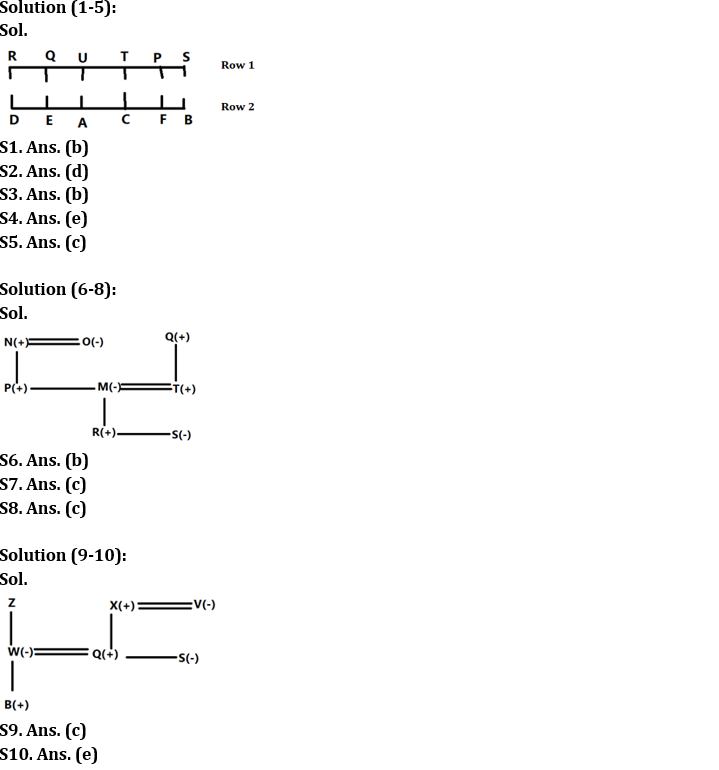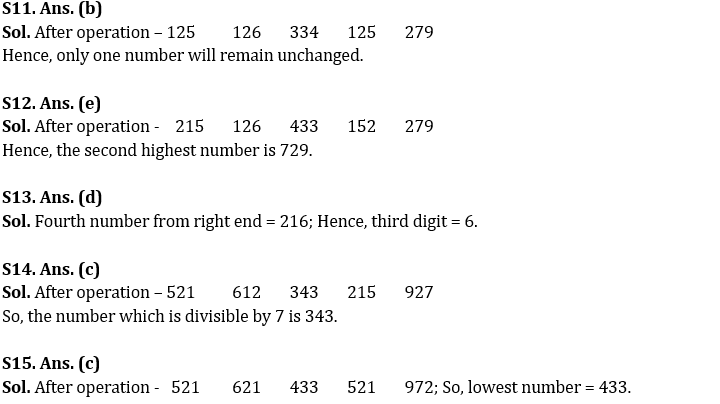Latest Banking jobs   »

# Reasoning Ability Quiz For IBPS RRB PO Clerk Prelims 2022- 26th July

Directions (1-5): Answer the questions based on the information given below.
12 persons sit in two parallel rows such that six persons sit in each row. P, Q, R, S, T and U sit in row 1 and face south while A, B, C, D, E and F sit in row 2 and face north. Persons in both rows face each other.
S sits opposite to B who is not adjacent to D and A. Q sits third to the right of P and neither of them sits at extreme end. D sits second to the left of A who is third person from one of the extreme ends. C sits immediate left of F. R sits second to the right of U.

Q1. Who among the following sits second to the left of C?
(a) A
(b) E
(c) Either E or B
(d) B
(e) Either A or E

Q2. Four of the following five are alike in a certain way and hence form a group. Who among the following doesn’t belong to that group?
(a) D
(b) B
(c) S
(d) U
(e) R

Q3. Who among the following sits fourth to the right of S?
(a) U
(b) Q
(c) R
(d) T
(e) None of the above

Q4. Who among the following sits opposite to R?
(a) E
(b) F
(c) C
(d) A
(e) D

Q5. How many persons sit between U and S?
(a) Four
(b) Three
(c) Two
(d) One
(e) None of the above

Directions (6-8): Study the given information and answer the related questions:
In a family of eight persons i.e., M, N, O, P, Q, R, S and T, there are two couples. Each couple is having only one son and one daughter. P is only son of N and sibling of M. M’s father-in-law is Q. S is the sister of R. R is the only son of M. O is the mother-in-law of T. One of them is single parent.

Q6. How is N related to S?
(a) Maternal grandmother
(b) Maternal grandfather
(c) Paternal grandmother
(d) Paternal grandfather
(e) None of the above

Q7. How is P related to T?
(a) Brother
(b) Son
(c) Brother-in-law
(d) Nephew
(e) None of the above

Q8. How many male members in the family?
(a) Three
(b) Four
(c) Five
(d) Either four or five
(e) None of the above

Directions (9-10): Study the given information and answer the related questions:
There are 7 members in family B, W, Q, S, Z, X, and V. There are two married couples in this family. Q is the son in law of Z. S is the sister-in-law of the W who is the only child of Z. X is the father-in-law of W who has only one male child. B is the nephew of S and V is the paternal grandmother of B. One of them is single parent.

Q9. Who is the wife of X?
(a) Z
(b) None of these
(c) V
(d) S
(e) W

Q10. How is Z related to S?
(a) Father
(b) Mother
(c) Uncle
(d) Aunt
(e) Can’t be determined

Directions (11-15): The following questions are based on three-digit numbers given below.

125 216 343 512 729

Q11. If all the digits within the number are arranged in ascending order, then how many numbers will remain unchanged?
(a) None
(b) One
(c) Two
(d) Three
(e) None of the above

Q12. If first and second digit of every number is interchanged within the number, then which will become second highest number?
(a) 125
(b) 216
(c) 343
(d) 512
(e) 729

Q13. Which of the following is third digit of fourth number from right end?
(a) 2
(b) 3
(c) 5
(d) 6
(e) None of the above

Q14. If the first and third digit of every number is interchanged within the number, then which of the following number will be divisible by 7?
(a) 125
(b) 216
(c) 343
(d) 512
(e) 729

Q15. If all the digit in the number is arranged in descending order, then which will become the lowest number?
(a) 125
(b) 216
(c) 343
(d) 512
(e) 729

Solutions#### Congratulations!Incorrect details? Fill the form again here

•Quantitative Aptitude Quiz For IBPS RRB ...
•Reasoning Ability Quiz For IBPS RRB PO C...
•Quantitative Aptitude Quiz For IBPS RRB ...
•Reasoning Ability Quiz For IBPS RRB PO C...
•Quantitative Aptitude Quiz For IBPS RRB ...
•Reasoning Ability Quiz For IBPS RRB PO C...